# DAV Class 3 Maths Chapter 12 Worksheet 3 Solutions

The DAV Maths Book Class 3 Solutions and DAV Class 3 Maths Chapter 12 Worksheet 3 Solutions of Geometry offer comprehensive answers to textbook questions.

## DAV Class 3 Maths Ch 12 WS 3 Solutions

Question 1.
Identify the following as line, line segment or ray.Solution: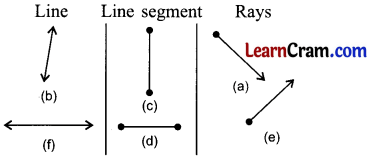Question 2.
Mark three points on the line segment AB. Also name them.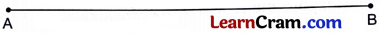Solution:Question 3.
In the given line segment, how many points are marked between-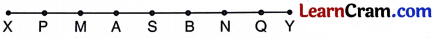(a) X and Y
Solution:
7

(b) P and B
Solution:
3Question 4.
How many line segments are there in each of the following?

(a)Solution:
4

(b)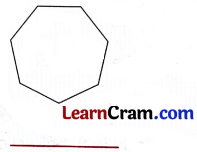Solution:
7

(c)Solution:
5

Question 5.
Name all the line segments in the following diagrams:

(a)Solution:
PQ, QR, RS

(b)Solution:
AB, BC, CD, DA, AC

(b)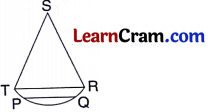Solution:
TS, SR, TR, PQQuestion 6.
Look at the figure and name-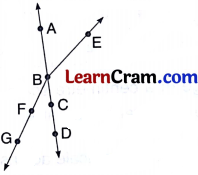(a) Any five points
Solution:
A, B, C, D, E

(b) One line
Solution:
AB

(c) Two rays
Solution:
BE, BA

(d) Two line segments
Solution:

Question 7.
Give any two examples of each of the following:

(a) Point
Solution:

1. Pointed end of the needle.
2. Pointed end of a pen.

(b) Line segment.
Solution:

1. Top of square table.
2. Comer of Blackboard.### DAV Class 3 Maths Chapter 12 Worksheet 3 Notes

Basic Geometry

Point:
A dot on paper with sharp pencil is a point. A point determines a location.

Line Segment:
A line segment has a definite length which can be measured.represent a line segment.

Line:
A line is a straight path which extends endlessly in both directions. A line contains countless number of points.The arrow heads show that a line extends endlessly in both direction.